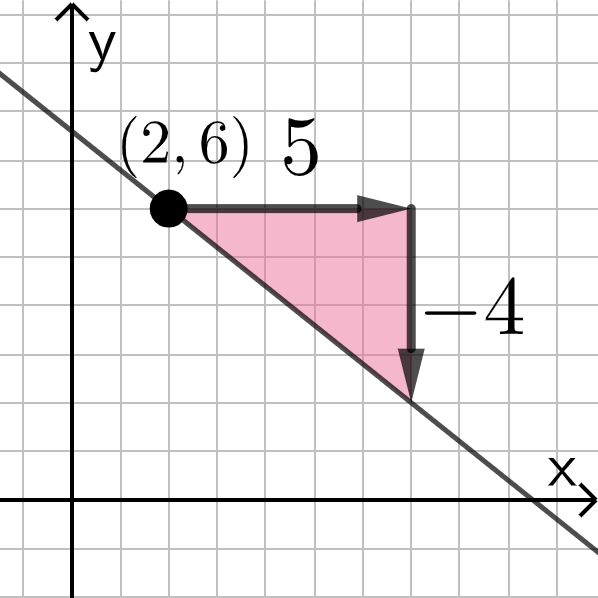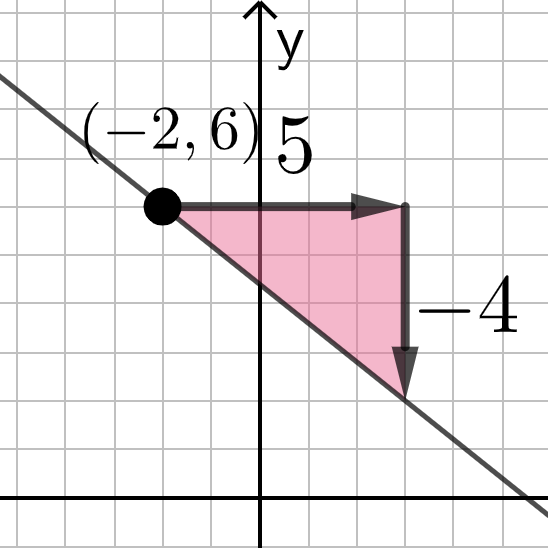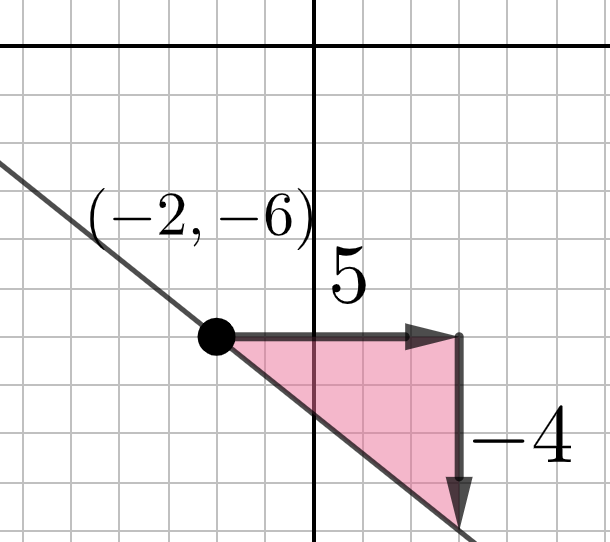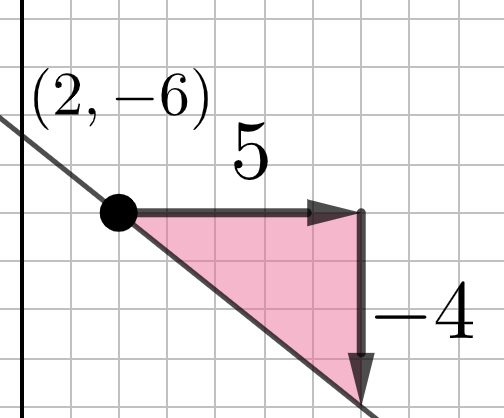Point-Slope Form: Quick Intro

The LARGE POINT (left) and sliders (right) are moveable. Interact with this app for a few minutes. Then answer the questions that follow.

What do you notice here? Be specific!

What is the equation of the line shown here?Check all that apply

What is the equation of the line shown here?Check all that apply

What is the equation of the line shown here?Check all that apply

What is the equation of the line shown here?Check all that apply

Through which point does the line pass through?

Check all that apply